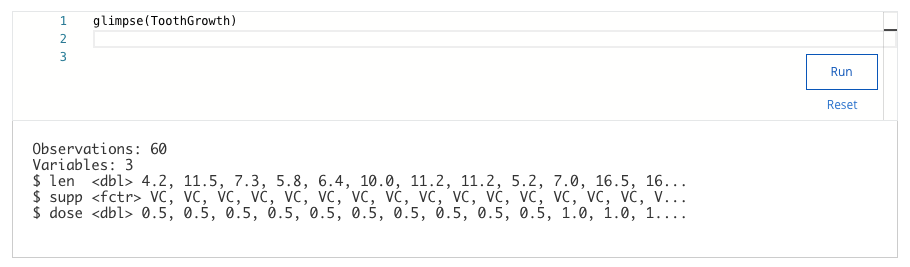## Week 3 – Working with data in R – Shuffle Q/A 3

• 3
• 10
• 2
• 6

#### 38. You are working with the ToothGrowth dataset. You want to use the glimpse() function to get a quick summary of the dataset. Write the code chunk that will give you this summary.• 2
• 3
• 60
• 1

#### 39. A data analyst is working with a data frame named customers. It has separate columns for area code (area_code) and phone number (phone_num). The analyst wants to combine the two columns into a single column called phone_number, with the area code and phone number separated by a hyphen. What code chunk lets the analyst create the phone_numbercolumn?

• unite(customers, “phone_number”, area_code, sep=”-”)
• unite(customers, “phone_number”, area_code, phone_num, sep=”-”)
• unite(customers, “phone_number”, area_code, phone_num)
• unite(customers, area_code, phone_num, sep=”-”)

• mean()
• max()
• cor()
• min()

#### 41. A data analyst is studying weather data. They write the following code chunk:bias(actual_temp, predicted_temp)What will this code chunk calculate?

• The average difference between the actual and predicted values
• The maximum difference between the actual and predicted values
• The total average of the values
• The minimum difference between the actual and predicted values

• str()
• mutate()
• colnames()

#### 43. A data analyst is using the unite() function to combine two columns into a single column. What does the sep parameter of the unite() function represent?

• The strings to place between each column
• The vector of columns to join into the final column
• The data frame that is the target of the operation
• The name of the final column formed from the original columns

• library()
• colnames()